KSEEB Solutions for Class 10 Maths Chapter 7 Coordinate Geometry Ex 7.4

Students can Download Class 10 Maths Chapter 7 Coordinate Geometry Ex 7.4 Questions and Answers, Notes Pdf, KSEEB Solutions for Class 10 Maths helps you to revise the complete Karnataka State Board Syllabus and score more marks in your examinations.

Karnataka State Syllabus Class 10 Maths Chapter 7 Coordinate Geometry Ex 7.4

Question 1.
Determine the ratio in which the line 2x + y – 4 = 0 divides the line segment joining the points A(2, – 2) and B(3, 7).
Let P(x1, y1) be common point of both lines and divide the line segment joining A(2, – 2) and B (3, 7) in ratio K : 1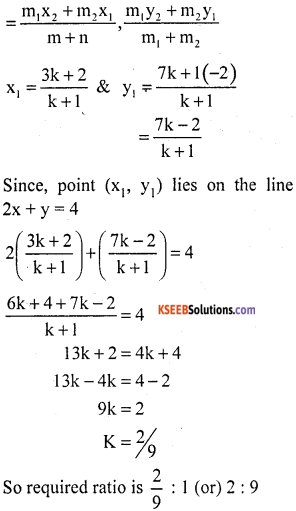Question 2.
Find a relation between x and y if the points (x, y), (1, 2) and (7, 0) are collinear.
Given point are A(x, y), B(1, 2) & C(7, 0) These points will be collinear if the area of the triangle formed by them is zero.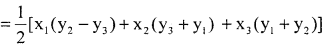0 = $$\frac{1}{2}$$[x(2 – 0)+1(0 – y)+7(y – 2)]
0 = x × 2 + 1 × – y + 7y – 14
0 = 2x – y + 7y – 14
2x + 6y – 14 = 0 divide by 2
x + 3y – 7 = 0
It is the relation between x & y

Question 3.
Find the centre of a circle passing through the points (6, – 6), (3, – 7) and (3, 3).
Let A → (6,- 6), B → (3,- 7) and C → (3, 3)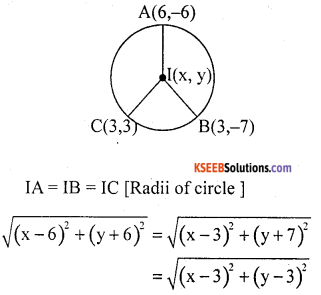Squaring
(x – 6)2 + (y + 6)2 = (x – 3)2 + (y + 7)2
x2 – 12x + 36 +y2 +12y + 36
= x2 – 6x + 9 + y2 +14y + 49
x2 – x2 + y2 – y2 – 12x +6x + 12y – 14y + 72 – 58 = 0
– 6x – 2y = – 14 devide by – 2
3x + y = 7 → (1)
(x – 3)2 +(y + 7)2 = (x – 3)2 +(y – 3)2 (y + 7)2 = (y – 3)2
y2 +49 + 14y = y2 + 9 – 6y
y2 – y2 + 14y + 6y = 9 – 49
20y = – 40
y = – 2
Putting y = – 2 in eqn (1) 3x + y = 7
3x – 2 = 7
3x = 7 + 2
3x = 9
x = $$\frac{9}{3}$$ = 3
x = 3
Thus I(x, y) → (3, – 2)
Hence, the centre of a circle is (3, – 2)

Question 4.
The two opposite vertices of a square are (- 1, 2) and (3, 2). Find the coordinates of the other two vertices.
Let ABCD is a square where two opposite vertices are A(- 1, 2) & C(3,2)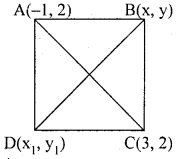AB = BC = CD = AD [ABCD is a square]
AB = BC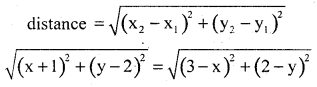Squaring both side
(x +1 )2 + (y – 2)2 = (3 – x )2 + (2 – y )2
x2 + 2x + 1 + y2 – 4y + 4
= 9 + x2 – 6x + 4 + y2 – 4y
2x + 6x – 4y + 4y = 13 – 5
8x = 8
x = $$\frac{8}{8}$$ = 1
x = 1
In ∆ ABC [B_ =90°x2 + 1 + 2x + y2 + 4 – 4x + 9 + x2
– 6x + 4 + y2 – 4y = (4)2
2x2 – 4x + 2y2 – 8y + 18 = 16
2x(1)2 – 4(1) + 2y2 – 8y + 18 – 16 = 0
2 – 4 + 2y2 – 8y + 2 = 0
2y2 – 8y = 0
2y(y – 4) = 0
2y = 0 & y – 4 = 0
y = 0 & y = 4
Hence, the other two vertices are (x, y) &(x1, y1) are (1, 0) & (1, 4)Question 5.
The Class X students of a secondary school in Krishinagar have been allotted a rectangular plot of land for their gardening activity. Sapling of Gulmohar are planted on the, boundary at a distance of 1m from each other. There is a triangular grassy lawn in the plot as shown in the Fig.7.4 The students are to sow seeds of flowering plants on the remaining area of the plot.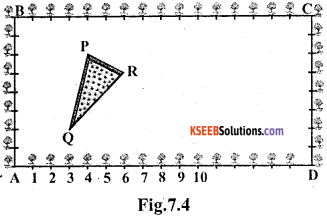(i) Taking A as origin,find the coordinates of the vertices of the triangle.
(ii) What will be the coordinates of the vertices of ∆ PQR if C is the origin? Also calculate the areas of the triangles in these cases. What do you observe?
(i) Taking A as origin then AD is x – axis and AB is y – axis.
Co-ordinates of P, Q and R are
P → (4, 6), Q → (3, 2) & R → (6, 5)
area of ∆ PQR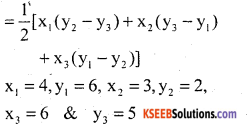area of ∆ PQR
= $$\frac{1}{2}$$[4(2 – 5) + 3(5 – 6) + (6 – 2)]
= $$\frac{1}{2}$$[4 × – 3 + 3 × – 1 + 6 × 4]
= $$\frac{1}{2}$$[- 12 – 3 + 24]
area of ∆ PQR
= $$\frac{1}{2}$$[- 15 + 24] = 9/2 sq units

(ii) Taking C as origin then CB is x – axis & CD is y – axis.
P(x1, y1) = (12, 2), Q(X2, y2) = (13, 6), and R(x3, y3) = (10, 3),
x1 = 12, y1 = 2, x2 = 13, y2 = 6, x3 = 10 & y3 = 3
area of ∆ PQR
= $$\frac{1}{2}$$[12(6 – 3) + 13(3 – 2) + 10(2 – 6)]
= $$\frac{1}{2}$$[12 x 3 + 13 x 1 + 10 x – 4]
= $$\frac{1}{2}$$[36 + 13 – 40]
= $$\frac{1}{2}$$[49 – 40] = 9/2 Sq units
Hence we observed that area of ∆ remains same in both case.

Question 6.
The vertices of a ∆ ABC are A(4, 6), B(1, 5) and C(7, 2). A line is drawn to intersect sides AB and AC at D and E respectively, such that AD/AB = AE/ AC = 1/4 Calculate the area of the ∆ ADE and compare it with the area of ∆ ABC. (Recall Theorem 6.2 and Theorem 6.6).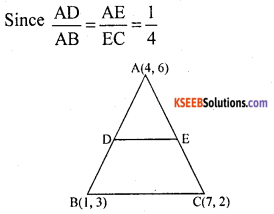D and E divide AB and AC respectively in the ration 1 : 3
Section Formula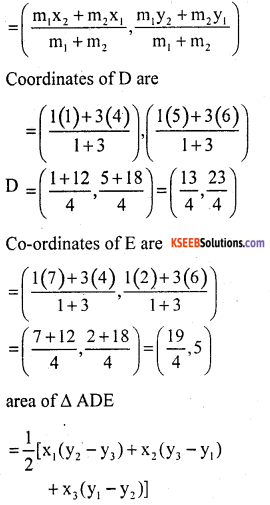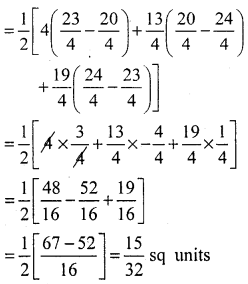area of ∆ ABC
= $$\frac{1}{2}$$[4(5 – 2) + 1(2 – 6) + 7(6 – 5)]
= $$\frac{1}{2}$$[4 × 3 + 1 × – 4 + 7 × 1]
= $$\frac{1}{2}$$[12 – 4 + 7]
= $$\frac{1}{2}$$ × [19 – 4]
= $$\frac{15}{2}$$sq units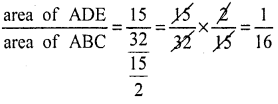area of ADE : area of ABC =1:16Question 7.
Let A (4,2), B(6, 5) and C(1, 4) be the vertices of ∆ ABC.
(i) The median from A meets BC at D. Find the coordinates of the point D.
(ii) Find the coordinates of the point P on AD such that AP : PD = 2 : 1
(iii) Find the coordinates of points Q and R on medians BE and CF respectively such that BQ : QE = 2:1 and CR : RF = 2:1.
(iv) What do yo observe?
[Note : The point which is common to all the three medians is called the centroid and this point divides each median in the ratio 2:1.]
(v) If A(x1, y1), B(x2, y2) and C(x3, y3) are the vertices of ∆ ABC, find the coordinates of the centroid of the triangle.
(i)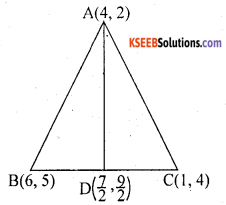median AD of the triangle ABC divide the side BC into two equal parts.
Therefore D is the mid – point of side BC Co-ordinates of mid Point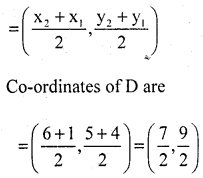(ii) Form equation AP : PD = 2 : 1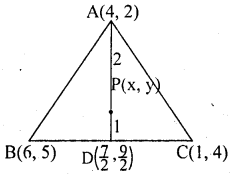Section Formula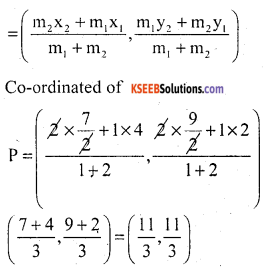(iii) BQ : QE = 2 : 1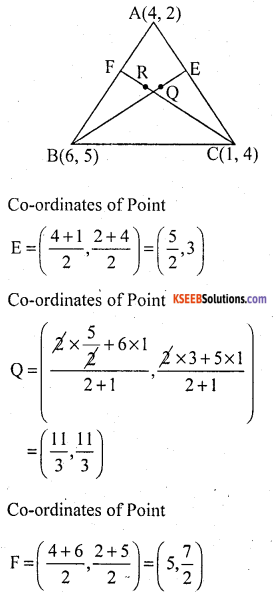co-ordinates of
$$\frac{A D}{A B}=\frac{A E}{E C}=\frac{1}{4}$$

(iv) The co-ordinates P, Q, & R are same (11/3, 11/3) All these points represents the same point which is called centriod.

(v) Point 0 is the centroid and AD is the midian. D is the mid point of BC and point 0 divide the AD into 2 : 1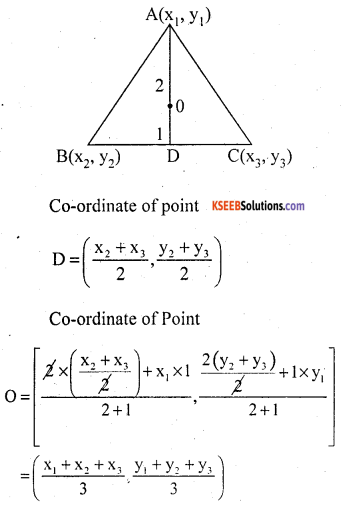Question 8.
ABCD is a rectangle formed by the points A(- 1, – 1), B(- 1, 4), C(5, 4) and D(5, – 1). P, Q, R and S are the mid-points of AB, BC, CD and DA respectively. Is the quadrilateral PQRS a square? a rectangle? or a rhombus? Justify your answer.
Given: A → (- 1, – 1), B → (- 1, 4), C → (5, 4) & D → (5, – 1) Co-ordinates of midpoint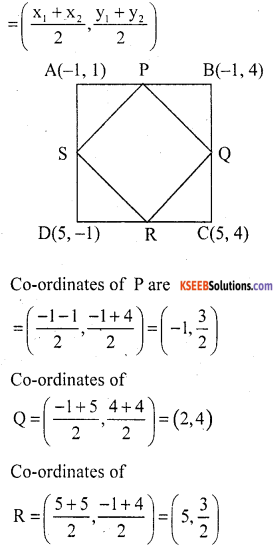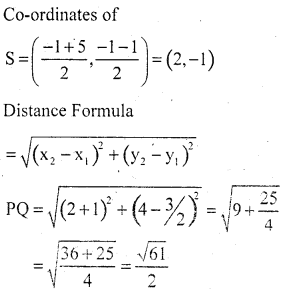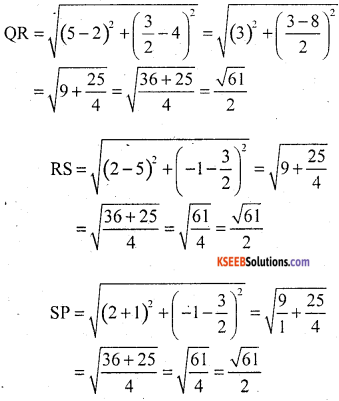∴ PQ = QR = RS = SP
∴ PQRS is either square (or) Rhomrus
Diagonal PR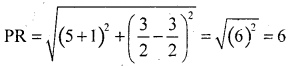and Diagonal QS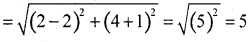PR ≠ QS
∴ Diagonal are not equal
Therefore, PQRS is a rhombus.